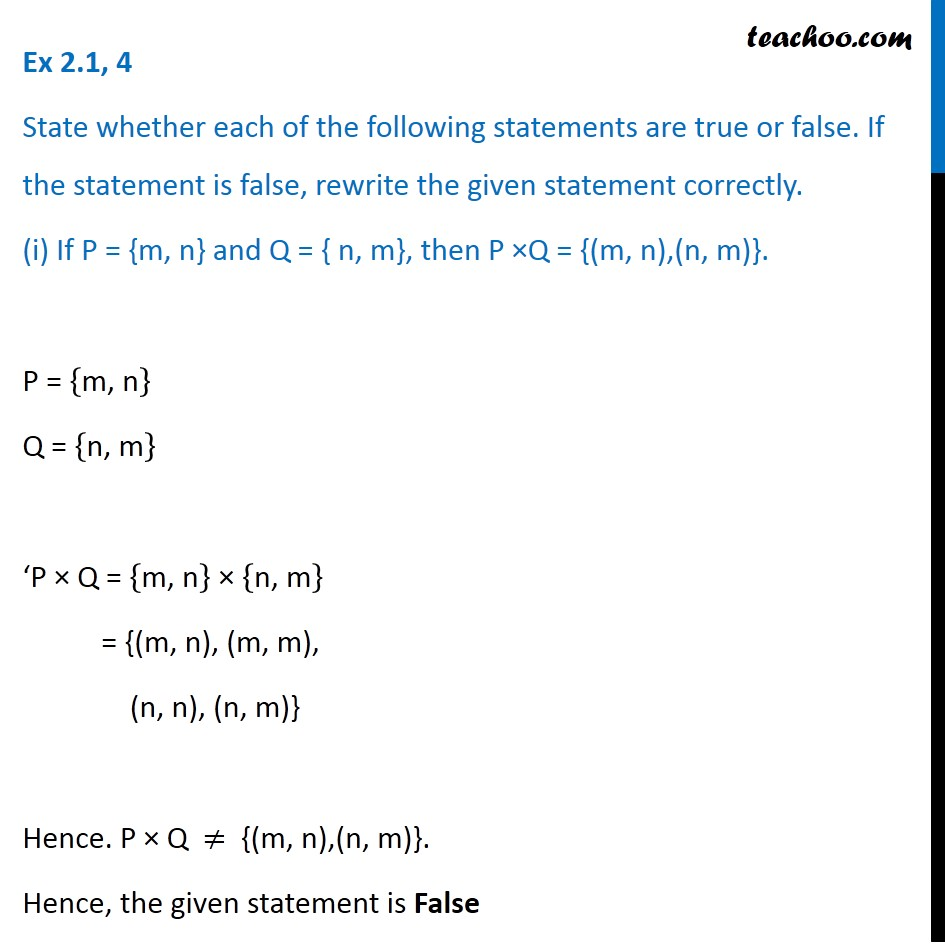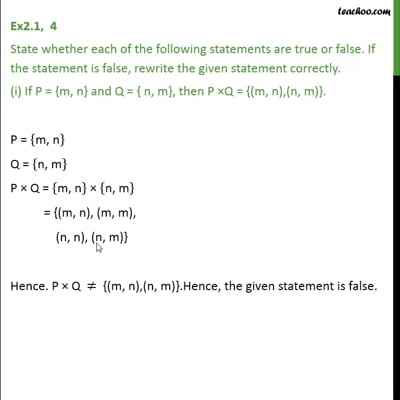Finding Cartesian Product

Chapter 2 Class 11 Relations and Functions
Concept wiseThis video is only available for Teachoo black users

Introducing your new favourite teacher - Teachoo Black, at only ₹83 per month

### Transcript

Ex 2.1, 4 State whether each of the following statements are true or false. If the statement is false, rewrite the given statement correctly. (i) If P = {m, n} and Q = { n, m}, then P ×Q = {(m, n),(n, m)}. P = {"m, n" } Q = {"n, m" } ‘P × Q = {"m, n" } × {"n, m" } = {(m, n), (m, m), (n, n), (n, m)} Hence. P × Q ≠ {(m, n),(n, m)}. Hence, the given statement is False The correct statement is If P = {m, n} and Q = { n, m}, then P × Q = {(m, n), (m, m), (n, n), (n, m)}